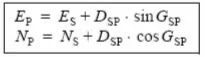How to calculate the coordinates of a vector ₪ 2.2 Calculation of Cartesian coordinates from a bearing

# How to calculate the coordinates of a vector ₪ 2.2 Calculation of Cartesian coordinates from a bearing

In topography, it is very common to know a point S (ES , NS) and to seek the coordinates of a point P visible from S .

We say that P is radiated from S if we can measure the horizontal distance DSP znf thr bearing GSP. Whatever the quadrant, we can then calculate the coordinates of the point P by the following formulas :Exercise n°2 :
S (680 379,84 ; 210 257,06) is given in Lambert coorfinate (m), calculate the coorinates of such that : DSP = 45,53 m and GSP = 172,622 gon.